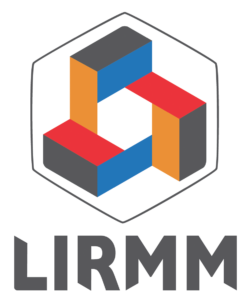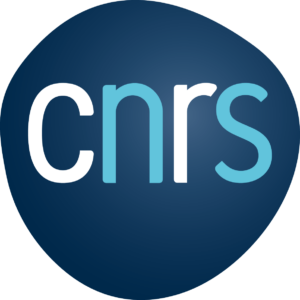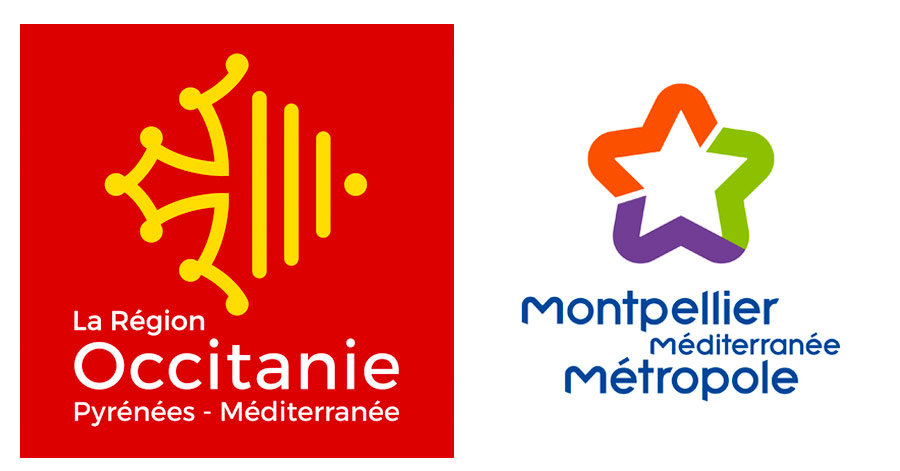\documentclass[11pt]{article}
\usepackage{latexsym,amsthm,amsmath,amssymb}
\usepackage[utf8x]{inputenc}

\newtheorem{theorem}{Theorem}
\newtheorem{lemma}{Lemma}

\pagestyle{empty}
\setlength{\textheight}{22cm}
\setlength{\topmargin}{-1cm}
\setlength{\textwidth}{165mm}
\setlength{\oddsidemargin}{-3mm}
\setlength{\unitlength}{1mm}

%\renewcommand{\refname}{\small References}
% References}}

\renewcommand{\title}{{\noindent\bf\Large #1}}
\newcommand{\speaker}{\noindent\underline{\bf #1} }
\newcommand{\coauthor}{{\noindent\bf#1 }}
\newcommand{\affiliation}{{\noindent\bf — #1 }}

%%%%%%%%%%%%%%%%
%%%%%%%%%%%%%%%%
%Put here the package you need, new commands, …
% Avoid non standard stuff if possible …

%%%%%%%%%%%%%%%%
%%%%%%%%%%%%%%%%

\begin{document}
%4 pages max !!

\vspace{2ex}

% The speaker (we will explicitly ask this information later, so at this point the choice does not need to be final)
\speaker{Alexander Grothendieck}
\affiliation{Université de Montpellier, France}

%A coauthor, add as many as needed

%The order of authors is up to you.
%It will appear as you write it !

\vspace{2ex}

\begin{abstract}
Maximum of 1000 characters. Please cut and paste this abstract to fill the field “abstract” on EasyChair when submitting. This is going to be used for our abstract booklet at the conference.
\end{abstract}

\section{Introduction}

Present as you wish, for instance: this paper is about \cite{berge:60}, and goes way beyond.

\begin{lemma}\label{lem:main-lemma}
The Petersen graph has 10 vertices.
\end{lemma}

\begin{theorem}
The graph obtained by the disjoint union of two Petersen graphs has 20 vertices.
\end{theorem}
\begin{proof} If suffices to observe that the order of a graph is additive with respect to its connected components, apply Lemma~\ref{lem:main-lemma} twice, and make the corresponding calculation correctly.
\end{proof}

\section{Concluding remarks}

We think that this was an amazing paper.

%If you need a ref section, do it more or less like that:
\small
\begin{thebibliography}{1}
\bibitem{berge:60}
C.~Berge.
\newblock Les problèmes de coloration en théorie des graphes.
\newblock Technical report, Publ. Inst. Stat. Univ. Paris, 1960.
\end{thebibliography}
\end{document}